# Comparison bar chart with differences

Excel 365
In Excel, you can create a variety of different charts to compare two or more data sets. When comparing two data sets, charts such as butterfly, mirror, tornado, etc., are often used, where the differences in the data sets are not always clearly visible.

Therefore, when creating a comparison chart, in addition to displaying two sets of data, it is also necessary to display the difference between them to create what is called a plus-minus chart: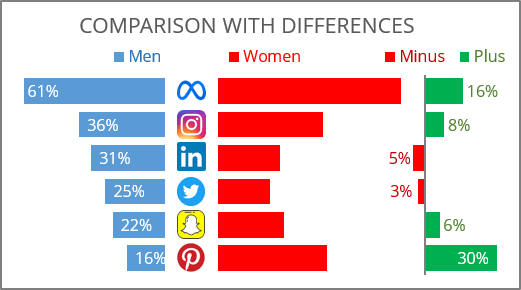To create a chart with differences like the one above (it will be created using the negative values, see more about other approaches), do the following:

### 1.   Prepare a data

For example, a chart is created for some survey data in a company about the use of the social media platform: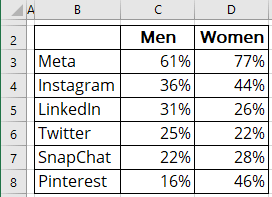1.1.   To center the chart, add the negative symbol (the symbol minus "-") to all values of the data series you prefer to see on the left (see how to quickly transform your data without using formulas).

1.2.   To see the space between data series (to put some icons or information such as ages), add a new column before the first data series and put some negative amount on it:

For example: -22%.

1.3.   Calculate differences using the following formulas:

• The Minus value is the difference between the first and the second datasets - if the second dataset is greater than the first,
• The Plus amount is the difference between the first and the second datasets - if the first dataset is greater than the second: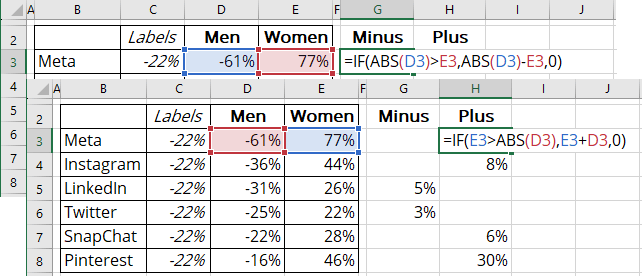where the ABS (<number>) function returns the absolute value of the <number> (the absolute value is the number without the minus).

Note: To see only valuable amounts and don't see zeros, format the cells using the following conditional formatting: [>0] #0%;;

1.4.   Calculate the widths for columns as a maximum of the values of this column:

• Width of the right column (in this example, = MAX (E3:E8) = 77%),
• Width of the Minus column (in this example, = MAX (G3:G8) = 5%).

1.5.   Add a new column after the data series to create a space between the data series and calculated differences with the following formula:

= maximum of the second dataset values + maximum of the Minus values + gap - the value of the second dataset and the Minus value,

where the gap is some amount that is defined for the space between columns (in this example, 10%):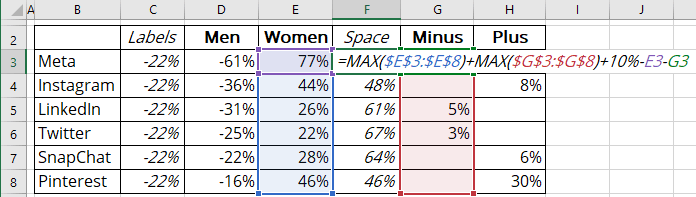### 2.   Create a chart

2.1.   Select the data range (in this example, B2:H8).

2.2.   On the Insert tab, in the Charts group, choose the Insert Column or Bar Chart button: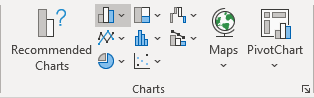From the Insert Column or Bar Chart dropdown list, under 2-D Bar, select Stacked Bar: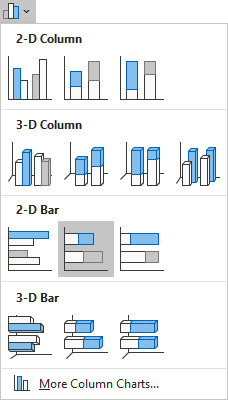Excel creates a simple stacked bar chart: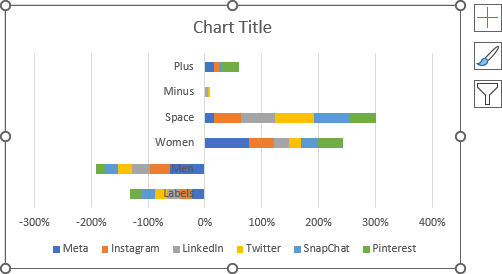2.3.   Switch rows and columns by doing one of the following:

• On the Chart Design tab, in the Data group, click the Switch Row/Column button: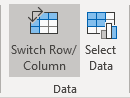• Right-click on the chart plot area and choose Select Data... in the popup menu: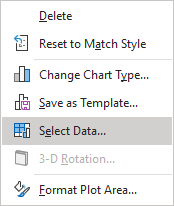In the Select Data Source dialog box, click the Switch Row/Column button: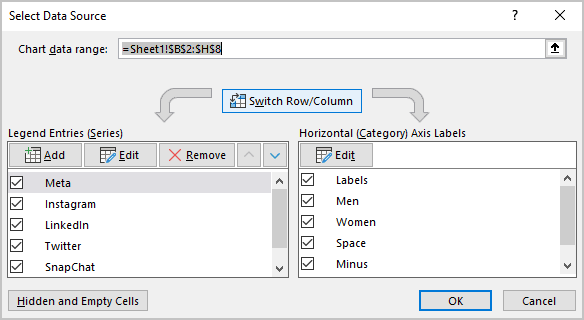Excel changes the chart: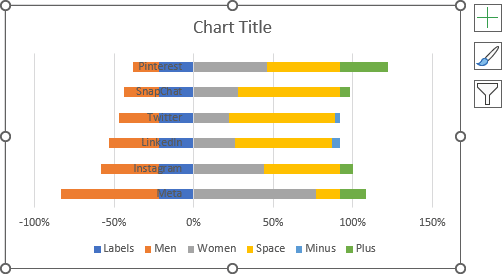### 3.   Format the vertical axis

3.1.   Right-click on the vertical axis, then click the Format Axis... in the popup menu: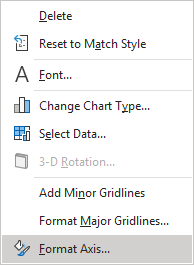3.2.   On the Format Axis pane, on the Axis Options tab:

• In the Axis Options section:
• Optionally, under Horizontal axis crosses, select the At maximum category option,
• At the end of the section, check the Categories in reverse order checkbox: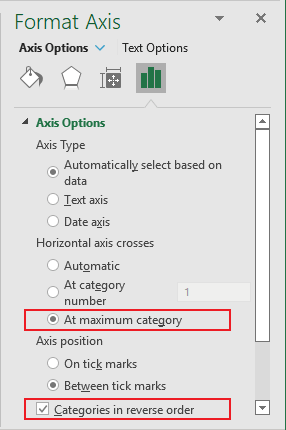• In the Labels section, from the Label Position dropdown list, select Low: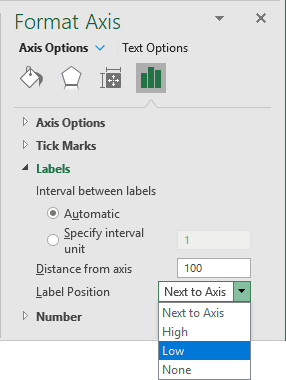### 4.   Format the horizontal axis

4.1.   Right-click on the horizontal axis and choose Format Axis... in the popup menu.

4.2.   On the Format Axis pane, on the Axis Options tab, in the Axis Options section:

• Under Bounds, type the appropriate value in the Minimum and Maximum fields.

In this example, -0.85 and 1.25.

• Under Vertical axis crosses, type the following value:

= maximum values from the second dataset + maximum values from the second dataset + the space between columns (in this example, 77% + 5% + 10%, see step 1.4).

In this example, 92%: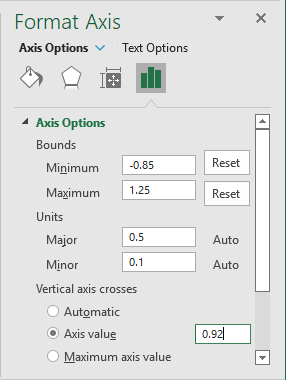### 5.   Format the data series

5.1.   Right-click on the first data series and choose Format Data Series... in the popup menu: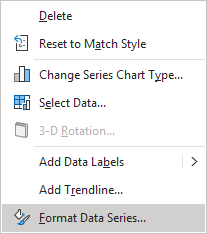5.2.   On the Format Data Series pane, on the Fill & Line tab:

• For every data series, select the appropriate filling option (see more about filling options in Microsoft).
• For the empty data series:
• In the Fill section, select the No fill option,
• In the Border section, select the No line option: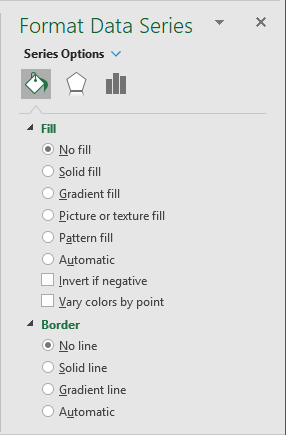5.3.   Optionally, on the Format Data Series pane, on the Series Options tab, in the Gap Width field, choose the value you like. For example, 50%: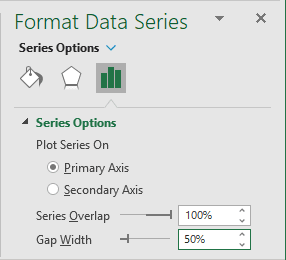### 6.   Add and format data labels

6.1.   Add the labels (see how to add data labels for more details).

6.2.   Right-click on any data labels for negative data series, then select Format Data Labels... in the popup menu: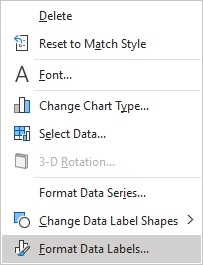6.3.   On the Format Data Labels pane, on the Label Options tab, in the Number group:

• From the Category dropdown list, select the Custom item,
• In the Format Code field, type the conditional formatting code (see more about conditional formatting):

<format for the positive value>; <format for the negative value>; <format for the zero>:

In this example, ;#0%;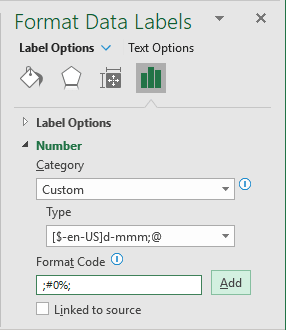### 7.   Format the chart

7.1.   Type the chart title, remove unnecessary labels from the legend, move the legend, hide the horizontal axis labels, then make any other adjustments you desire: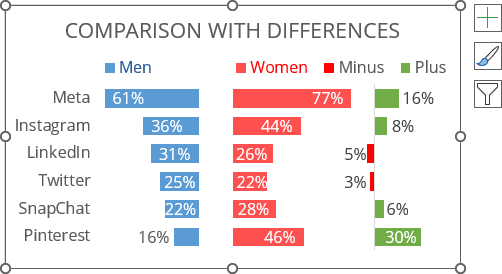7.2.   Add images to the middle empty data series, hide the vertical axis labels, and format the vertical axis line.

Make any other adjustments you desire.